# Linear Models

This section describes multivariate regression analysis using a variety of methods. When the data at hand is fat-tailed or cannot be transformed to be normally distributed, then the assumptions for ordinary least squares regression are violated. In such cases, we can use robust regression and ols-t regression. In other scenarios perhaps we are dealing with issues of multicollinearity or variable proliferation, then we have Lasso and Ridge to choose from.

## OLS Regression#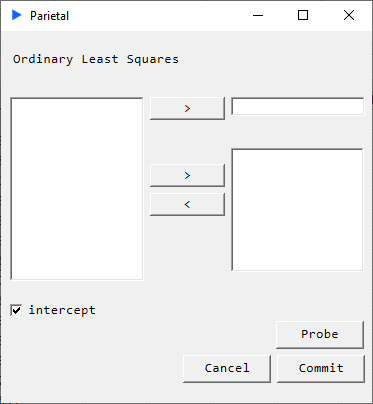##### Description#

Suppose we have the following system: $$Y = X \beta$$

where

$$X=\begin{bmatrix} x_{11} & x_{12} & \cdots & x_{1p}\\ x_{21} & x_{22} & \cdots & x_{2p}\\ \vdots & \vdots & \ddots & \vdots\\ x_{n1} & x_{n2} & \cdots & x_{np} \end{bmatrix} , \beta = \begin{bmatrix} \beta_1 \\ \beta_2 \\ \vdots \\ \beta_p \end{bmatrix} , Y = \begin{bmatrix} y_1 \\ y_2 \\ \vdots \\ y_n \end{bmatrix}$$

We solve the quadratic minimization problem given by: $$\mathrm{\hat{\beta} = \underset{\beta}{\operatorname{arg\min}}\ F(\beta)}$$

where the objective function $F$ is given by: $$\mathrm{ F(\beta) = \sum_{i=1}^n \biggl| y_i - \sum_{j=1}^p X_{ij}\beta_j\biggr|^2 = | y - X \beta |^2 }$$

We obtain: $$\mathrm{ \hat{\beta}= \left( X^{T} X \right)^{-1} X^{T} y }$$

Since matrix inversions are extremely high in time complexity, we use a QR decomposition to solve the system.

##### Returns#

Main regression table and model metrics:Goodness of fit measures and diagnostics: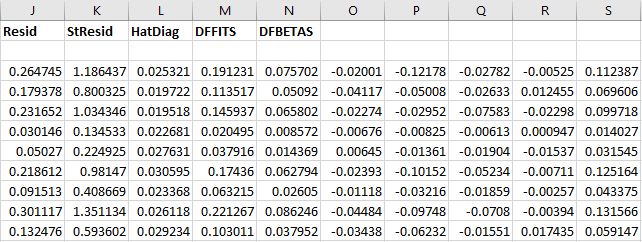• coef: coefficients
• serr: standard errors
• tstat: t-statistic
• pval: p-value
• rse: residual standard error
• dof: degrees of freedom
• rsq: r-squared
• fStat: F-statistic
• fProb: p-value for model
• Resid: model residuals
• StResid: model standardized residuals
• HatDiag: hat diagonal
• DFFITS: studentized influence on predicted values
• DFBETAS: studentized influence on coefficients

## OLS with t-distributed errors#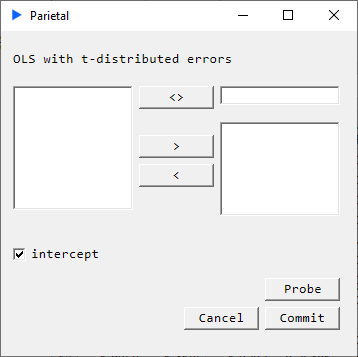##### Description#

We carry out a multivariate regression analysis but we assume that model errors are t-distributed.

$$y = \mathrm{X} \boldsymbol{\beta} + \boldsymbol{\epsilon}, \boldsymbol{\epsilon} \sim t(\mu, \sigma, \nu)$$

An iterative method known as iteratively reweighted least squares (IRLS) is carried out until the estimates converge to an acceptable tolerance.

$$\hat{\beta}^{(t+1)}=(X^{T}(W^{-1})^{t}X)^{-1}X^{T}(W^{-1})^{t}y$$

##### Returns#
• coef: coefficients
• serr: standard errors
• tstat: t-statistic
• pval: p-value
• rse: residual standard error
• dof: degrees of freedom
• rsq: r-squared
• fStat: F-statistic
• fProb: p-value for model
• Resid: model residuals
• StResid: model standardized residuals

## Robust Regression#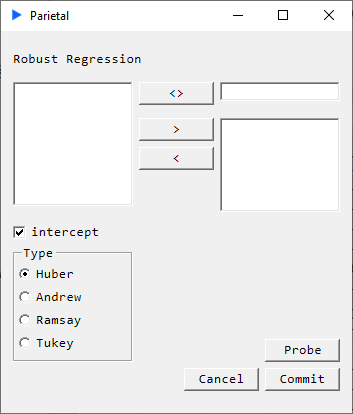##### Description#

The purpose of robust regression methods is to dampen the influence of outliers in data by specifying a weight function. A number of these weight functions have been proposed. We implement four of these:

• Huber
• Andrew
• Ramsay
• Tukey

An iterative method known as iteratively reweighted least squares (IRLS) is carried out until the estimates converge to an acceptable tolerance.

$$\mathrm{\hat{\beta}^{t+1}=(X^{\textrm{T}}(W^{-1})^{t}X)^{-1}X^{T}(W^{-1})^{t}y}$$

where

$$\mathrm{w_{i}^{t}= \begin{cases}\dfrac{\psi((y_{i}-x_{i}^{t}\beta^{t})/\hat{\tau}^{t})}{(y_{i} x_{i}^{t}\beta^{t})/\hat{\tau}^{t}} & {if (y_{i} \neq x_{i}^{\textrm{T}}\beta^{t})} \\ 1 & {if (y_{i}=x_{i}^{\textrm{T}}\beta^{t})} \end{cases}}$$

##### Returns#
• coef: coefficients
• serr: standard errors
• tstat: t-statistic
• pval: p-value
• rse: residual standard error
• dof: degrees of freedom
• rsq: r-squared
• fStat: F-statistic
• fProb: p-value for model
• Resid: model residuals
• StResid: model standardized residuals

## Ridge Regression and CV#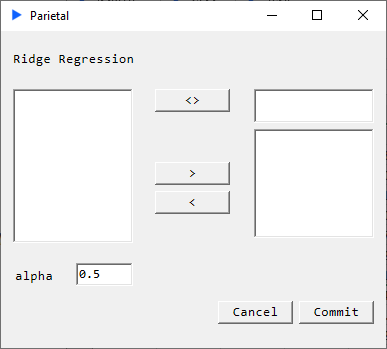##### Description#

Ridge regression is a form of penalized regression. The parameter that controls this penalty is $\alpha$ which can range from 0 (no penalty) to 1. The penalty prevents against a variable having an outsized coefficient compared to others.

$$\hat{\beta}_{ridge} = (X^T X + \lambda I_p)^{-1} X^T Y$$

We solve for coefficients that minimize:

$$\sum_{i=1}^n (y_i - \sum_{j=1}^p x_{ij}\beta_j)^2 + \lambda \sum_{j=1}^p \beta_j^2$$

##### Returns#
• coefficients
• MSE
• predicted values
##### Cross Validation Returns#
• best $\alpha$
• best model MSE
• coefficients
• predicted values
• $\alpha$ path
• regularization path
• MSE grid

## Lasso Regression and CV#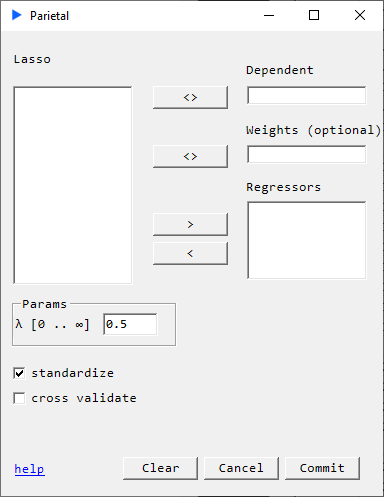Elastic Net combines $L_{1}$ and $L_{2}$ penalties. The two parameters that control this penalty are $\lambda \in [0, \infty)$ and $\alpha \in [0, 1]$.

$\alpha$ balances Ridge and LASSO penalties with $\alpha = 1$ being LASSO. We use coordinate descent for the updates.

$$\min_{(\beta_0, \beta) \in \mathbb{R}^{p+1}}\frac{1}{2N} \sum_{i=1}^N (y_i -\beta_0-x_i^T \beta)^2+\lambda \left[ (1-\alpha)||\beta||_2^2/2 + \alpha||\beta||_1\right],$$

where $\lambda \geq 0$ is known as the complexity parameter and $\alpha \in [0, 1]\$ is the ridge parameter.

If the cross validation option is checked, a 10-fold cross validation is performed. The $\lambda$ path used is generated using the method described by Hastie-Tibshirani .

##### Returns#
• $\alpha$
• $\lambda$
• MSE
• coefficients
• predicted values
##### Cross Validation Returns#
• $\alpha = 1$
• best model $\lambda$
• best model MSE
• coefficients
• predicted values
• $\lambda$ path
• regularization path
• MSE grid

 Regularization Paths for Generalized Linear Models via Coordinate Descent (Journal of Statistical Software - Jan 2010)

## Least Absolute Deviation Regression###### Description#

Where OLS regression seeks to minimize the L2 norm, least absolute deviation (LAD) regression seeks to minimize the L1 norm $S = \sum_{i=1}^n |y_i - f(x_i)|$ . We use iteratively weighted least squares (IRLS) to solve this problem. LAD is more resistant to outliers than OLS and is therefore typically considered a form of robust regression.

##### Returns#
• coef: coefficients
• serr: standard errors
• tstat: t-statistic
• pval: p-value
• rse: residual standard error
• dof: degrees of freedom
• rsq: r-squared